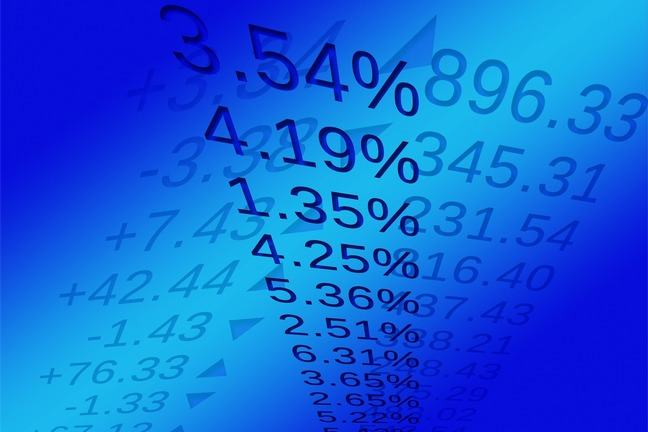2.7

## SOAS University of London# The risk of financial assets

What would happen to the riskiness of an asset, and to our measure of risk, if extreme returns became more likely?

We can examine this question by computing the expected return and standard deviation in an example. Before we do that, what do you think will be the result, if we increase the chances of extreme events?

We believe the returns on asset $A$ follow this probability distribution:

Probability Return: $R_A$
0.10 –3%
0.30 2%
0.50 8%
0.10 12%

The extreme returns –3% and 12% have a probability of 0.10 = 10% each; there is a probability of 30% that the return on the security is 2%; and a probability of 50% that the return is 8%. Let’s now compute the expected return and standard deviation using the formulas from the earlier step.

Remember we calculate the expected return by multiplying each possible return by the probability of the return occurring, and then adding these products together:

The expected return is $\mu_A = E(R_A) = 5.5\%$.

## What is its standard deviation?

We first see how much each possible return deviates from the expected return, and then square those differences, as shown in the following table:

Probability $R_A$ - $\mu_A$ $(R_A - \mu_A)^2$
0.10 –0.03 – 0.055 = –0.085 0.007225
0.30 0.02 –0.055 = –0.035 0.001225
0.50 0.08 – 0.055 = 0.025 0.000625
0.10 0.12 – 0.055 = 0.065 0.004225

We sum these squared deviations (with each squared deviation multiplied by the probability of the return occurring) to get the variance:

The variance is measured in terms of the returns squared. Therefore we take the square root of the variance to get the standard deviation, which is measured in terms of the returns themselves:

We can see that asset $A$ has an expected return of 5.5% and a standard deviation of 4.272%.

What would happen if the returns on the asset were to become “riskier”, with the extreme returns –3% and 12% becoming more likely?

Suppose the distribution of returns on the asset is now described by the following table:

Probability Return: $R_A$
0.20 –3%
0.20 2%
0.40 8%
0.20 12%

Can you see the lowest and highest returns are now more likely, each with a probability of 20% instead of 10%, and the middle returns are correspondingly less likely? Incidentally, have you noticed how the probabilities in the tables always add up to one? And the sum of the probabilities should not add up to more than one. Why is that?

The expected return $\mu_A$ is now:

The expected return is slightly smaller than it was before.

To compute the variance we look again at the difference between each return and the expected return, and square that difference:

Probability $R_A - \mu_A$ $(R_A - \mu_A)^2$
0.20 –0.03 – 0.054 = –0.084 0.007056
0.20 0.02 – 0.054 = –0.034 0.001156
0.40 0.08 – 0.054 = 0.026 0.000676
0.20 0.12 – 0.054 = 0.066 0.004356

The variance is:

and the standard deviation is:

In this second probability distribution we have increased the probability of observing the two extreme returns. The expected return of 5.4% is close to the expected return in the first case (5.5%). But the standard deviation of 5.276% is now higher (than 4.272%). The asset has become riskier, and our measure of risk reflects this.

Is that what you predicted?

Share your understanding by discussing this question in the comments section.